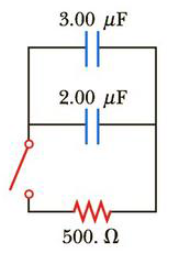Chapter 18, Problem 67AP

Chapter
Section
Textbook Problem

The given pair of capacitors in Figure P18.67 is fully charged by a 12.0-V battery. The battery is disconnected and the circuit closed. After 1.00 ms, how much charge remains on (a) the 3.00-μF capacitor? (b) The 2.00-μF capacitor? (c) What is the current in the resistor?Figure P18.67

(a)

To determine

The current in the 4.00V battery from the given circuit.

Explanation

Given Info: Apply the Kirchhoff’s junction rule and loop rule to the loop ‘gbcfg’ and ‘fcdef

Apply the junction rule at the point c in the given figure we get,

I1=I3I2                                                                        (1)

• I1, I2 and I3 is the current in the given circuit,

Apply kirchoff’s loop rule to the loop gbcfg.

8.00V+3.00I25.00I1=0

Rewrite the above relation,

5.00I13.00I2=8.00V                                                     (2)

Again apply kirchoff’s loop rule to the loop fcdef we get,

3.00I25.00I3+4.00V=0

Rewrite the above relation,

5.00I3+3.00I2=4.00V                                                    (3)

Substitute equation (1) into equation (2) will get,

I1=I3I25

(b)

To determine

The current in the 3.00Ω resistor.

(c)

To determine

The current passing through the 8.00 V batteries.

(d)

To determine

The current passing through the 3.00 V batteries.

(e)

To determine

The charge on the capacitor.

Still sussing out bartleby?

Check out a sample textbook solution.

See a sample solution

The Solution to Your Study Problems

Bartleby provides explanations to thousands of textbook problems written by our experts, many with advanced degrees!

Get Started